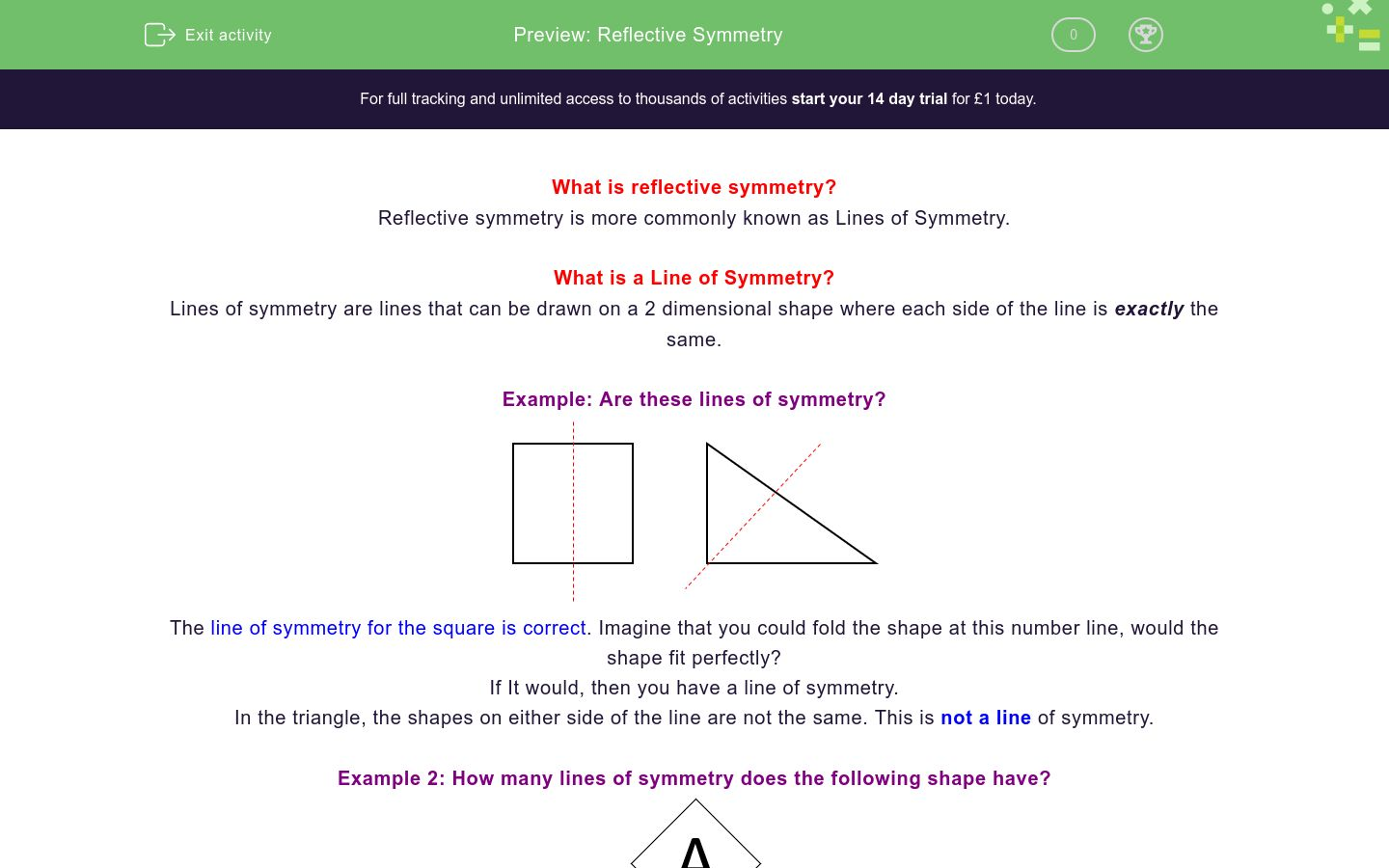# Reflective Symmetry

In this worksheet, students identify lines of symmetry.Key stage:  KS 4

GCSE Subjects:   Maths

GCSE Boards:   AQA, Eduqas, Pearson Edexcel, OCR

Curriculum topic:   Geometry and Measures, Basic Geometry

Curriculum subtopic:   Properties and Constructions, Conventions, Notations and Terms

Difficulty level:### QUESTION 1 of 10

What is reflective symmetry?

Reflective symmetry is more commonly known as Lines of Symmetry.

What is a Line of Symmetry?

Lines of symmetry are lines that can be drawn on a 2 dimensional shape where each side of the line is exactly the same.

Example: Are these lines of symmetry?The line of symmetry for the square is correct. Imagine that you could fold the shape at this number line, would the shape fit perfectly?

If It would, then you have a line of symmetry.

In the triangle, the shapes on either side of the line are not the same. This is not a line of symmetry.

Example 2: How many lines of symmetry does the following shape have?This has two elements. The square and the letter A.

A square has 4 lines of symmetry, the letter A has one line of symmetry.

When we have two elements, the lower number of lines of symmetry is the one we use.

In this case, we have 1 Line of Symmetry.Example 3: How many lines of symmetry does the following shape have?This one is actually easier than the last one, this is a regular hexagon (remember: regular means all the angles and sides are the same.)

When we have a regular shape, the number of lines of symmetry is the same as the number of sides so this shape has 6 lines of symmetryLines of symmetry are...

How many lines of symmetry does a square have?

1

2

3

4

How many lines of symmetry does a rectangle have?

1

2

3

4

A triangle can have a different amount of lines of symmetry depending on what type of triangle it is...

1

2

3

4

Match the geometric shape with the number of lines of symmetry it has.

## Column B

Rectangle
4
Equilateral triangle
3
Square
2
Isosceles trapezium
1

Is this a line of symmetry?Yes

No

Is this a line of symmetry?Yes

No

How many lines of symmetry does a regular octagon have?

How many lines of symmetry does this shape have?1

4

How many lines of symmetry does this shape have?• Question 1

Lines of symmetry are...

EDDIE SAYS
This is the very definition of a line of symmetry. The line has to be in a place on the shape that if you put a mirror on the line, the shape would appear the same. This is why Lines of Symmetry is also called Reflective Symmetry.
• Question 2

How many lines of symmetry does a square have?

4
EDDIE SAYS
A square has all the angles and sides the same. This means it is a regular shape. If we have a regular shape, we said in the intro that the number of lines of symmetry are the same as the number of sides for a regular shape.
• Question 3

How many lines of symmetry does a rectangle have?

2
EDDIE SAYS
It's a really common mistake to say a rectangle has 4 lines of symmetry. It has one down through the middle and one across the middle. If you folded a rectangle corner to corner, do the two sides line up?
• Question 4

A triangle can have a different amount of lines of symmetry depending on what type of triangle it is...

EDDIE SAYS
An equilateral triangle is a regular shape so it must have three. An isosceles can only be split one way (can you think where that will be) A scalene is the one people always forget about, this is the triangle where all the sides (and therefore angles) are different.
• Question 5

Match the geometric shape with the number of lines of symmetry it has.

## Column B

Rectangle
2
Equilateral triangle
3
Square
4
Isosceles trapezium
1
EDDIE SAYS
You could do this by a process of elimination. Which of the four shapes are regular (HINT: There's two of them) Then apply your knowledge of the shapes we have looked at to sort the other two.
• Question 6

Is this a line of symmetry?No
EDDIE SAYS
Is the shape above the line a reflection of the shape under the line?
• Question 7

Is this a line of symmetry?No
EDDIE SAYS
Is the shape above the line a reflection of the shape under the line?
• Question 8

How many lines of symmetry does a regular octagon have?

8
eight
EDDIE SAYS
There's a couple of hints in this question. Regular - The shape will have the same number of lines of symmetry as it does sides. Octagon - What does the prefix OCT- mean?
• Question 9

How many lines of symmetry does this shape have?1
EDDIE SAYS
There are two shapes here. The square has 4 lines of symmetry The triangle has 1. Which one of these will the combined shape have? It\'s always the lower one.
• Question 10

How many lines of symmetry does this shape have?2
two
EDDIE SAYS
There are two shapes here. The rectangle has 2 lines of symmetry and so does the X shape. They don't always have to be different.
---- OR ----

Sign up for a £1 trial so you can track and measure your child's progress on this activity.

### What is EdPlace?

We're your National Curriculum aligned online education content provider helping each child succeed in English, maths and science from year 1 to GCSE. With an EdPlace account you’ll be able to track and measure progress, helping each child achieve their best. We build confidence and attainment by personalising each child’s learning at a level that suits them.

Get started